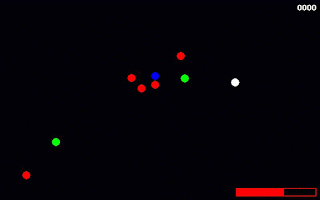## Posts

Showing posts from December, 2018

### DumbFire, a simple shooting gamePtEn< change language DumbFire is a very simple shooting game that I created with Python and pygame and you can find the code here. As of now, the game does not have a menu whatsoever nor it has any kind of instructions...
(btw, to play it use the WASD keys to move and the space bar to shoot; if the coloured balls hit you, you lose health; if you shoot them, you get health back)

I have been incredibly busy so I am not sure I will tidy this up any time soon but feel free to fork the repo in GitHub and to add different types of enemies and maybe some kind of power-up or whatnot. Actually, I would be very interested in hearing from you the answer to: if I could only add one single extra functionality to the game, what would that be? O jogo DumbFire é um jogo simplecíssimo de tiros que eu fiz com Python e com pygame. O código está no repo usual e convido-vos a fazerem uma cópia do mesmo para experimentarem alterar os tipos de inimigos, talvez juntar um ou outro power-up, etc. De momento…

### Building an 8-bit addition calculator with circuitsPtEn< change language I have a childhood friend that had very interesting toys... He used to play around with circuits, multimeters, LED lights, small batteries, etc. I loved going to his house and watch him play with all that. That is probably why I find circuits (in their basic form) very interesting: the current flowing and the logic gates operating on the circuits and whatnot.

Because of that, in this post I will show you how to build an 8-bit addition calculator just with circuits! For that I will be using logic.ly, a circuits simulator that I can run in my browser.

The best way to go about this is by starting with small components and then using a nice feature of logic.ly, which enables one to create "integrated circuits": it takes one circuit we created and transforms it into a single piece.

Of course that before anything else one must know how to add two numbers in binary. It is essentially the same as in with decimal numbers, except that $1 + 1 = 10$ now. To cre…

### Pocket maths: good rational approximations

PtEn< change language An obvious way of creating rational approximations for irrational numbers is by truncating its decimal expansion. For example, $3$, $3.1$ and $3.14$ are all rational approximations of $\pi$; as fractions, those approximations would be written $3$, $\frac{31}{10}$ and $\frac{314}{100}$.

Notice how $\frac{314}{100}$ has $100$ as the denominator and yet only produces an approximation correct up to two decimal places.

Claim: by using continued fractions one can obtain better rational approximations for irrational numbers.

Method: if $x$ is an irrational number, instead of truncating its decimal expansion, we can truncate its continued fraction.
Taking $\pi$ as an example, we have $$\pi = 3 + \frac1{7 + \frac1{15 + \cdots}}$$ and by taking $$\pi \approx 3 + \frac17 = \frac{22}{7}$$ we get the approximation $\pi \approx 3.14285\cdots$: it is correct up to two decimal places just as $\frac{314}{100}$, but $7$ is a much smaller denominator than $100$. (And al…